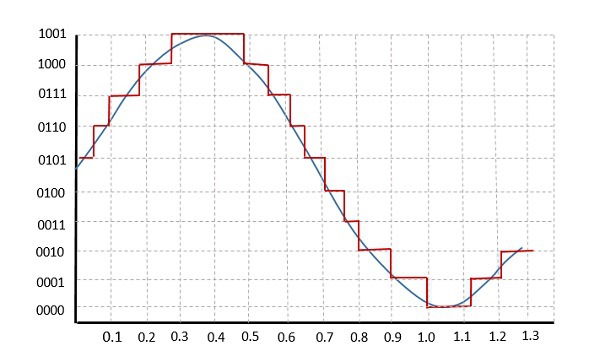# Modulation Techniques

There are few modulation techniques which are followed to construct a PCM signal. These techniques like sampling, quantization, and companding help to create an effective PCM signal, which can exactly reproduce the original signal.

## Quantization

The digitization of analog signals involves the rounding off of the values which are approximately equal to the analog values. The method of sampling chooses few points on the analog signal and then these points are joined to round off the value to a near stabilized value. Such a process is called as Quantization.

The quantizing of an analog signal is done by discretizing the signal with a number of quantization levels. Quantization is representing the sampled values of the amplitude by a finite set of levels, which means converting a continuous-amplitude sample into a discrete-time signal.

The following figure shows how an analog signal gets quantized. The blue line represents analog signal while the red one represents the quantized signal.Both sampling and quantization results in the loss of information. The quality of a Quantizer output depends upon the number of quantization levels used. The discrete amplitudes of the quantized output are called as representation levels or reconstruction levels. The spacing between two adjacent representation levels is called a quantum or step-size.

## Companding in PCM

The word Companding is a combination of Compressing and Expanding, which means that it does both. This is a non-linear technique used in PCM which compresses the data at the transmitter and expands the same data at the receiver. The effects of noise and crosstalk are reduced by using this technique.

There are two types of Companding techniques.

### A-law Companding Technique

• Uniform quantization is achieved at A = 1, where the characteristic curve is linear and there is no compression.

• A-law has mid-rise at the origin. Hence, it contains a non-zero value.

• A-law companding is used for PCM telephone systems.

• A-law is used in many parts of the world.

### µ-law Companding Technique

• Uniform quantization is achieved at µ = 0, where the characteristic curve is linear and there is no compression.

• µ-law has mid-tread at the origin. Hence, it contains a zero value.

• µ-law companding is used for speech and music signals.

• µ-law is used in North America and Japan.

## Differential PCM

The samples that are highly correlated, when encoded by PCM technique, leave redundant information behind. To process this redundant information and to have a better output, it is a wise decision to take predicted sampled values, assumed from its previous outputs and summarize them with the quantized values.

Such a process is named as Differential PCM technique.